Next: 3.3 Extension of the Up: 3.2 Extension of Neural Previous: 3.2.2 Alternative distance measure

## 3.2.3 Simulations

The operation of the algorithm is demonstrated on two synthetic ring-ling-square distributions. The data points in these distributions are uniformly distributed over the area of a ring, a line, and a square. The outer radius of the ring equals 1.0. The first variant consists of 850 data points (figure 3.2); the second is much more sparse and contains only 85 points (figure 3.3).

In this section, all tests used the same parameters. Ten units were used. For each, two principal components were extracted. The further training parameter settings were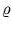(0) = 1.0,(tmax) = 0.001,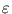(0) = 0.5,(tmax) = 0.001, and tmax = 30 000. The quality of a fitted mixture model was evaluated by computing log-likelihoods. As mentioned before, each unit can be interpreted as a local probability density, p(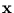| j) = (2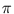)-d/2exp(- Ej()/2) (appendix C.1). These local densities allow the definition of a total density underlying the data distribution. It remains to define the priors, the weights of the local units. The prior P(j) is set equal to the fraction of data points assigned to unit j. This assignment is obtained using hard-clustering based on (3.2). Given the priors, the log-likelihood per pattern is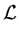=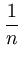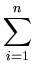ln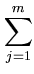P(j)p(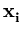| j) . (3.11)

To compute p(| j), NGPCA-constV uses (3.2) for Ej() and (3.10) to obtain the prior P(j), since the modification just affects the competition between units.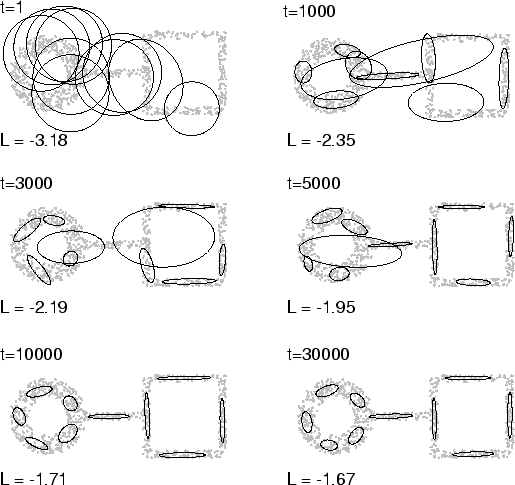The first test, using NGPCA, shows the incremental adjustment of the ellipses to the ring-line-square distribution with 850 points (figure 3.2). Here, only one training cycle is shown, but the performance was stable over different training cycles. In ten cycles, the final fitted model resembled the one shown in figure 3.2, and the final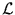ranged between -1.669 and -1.665. NGPCA-constV resulted in similar fitted models; here, the finalranged between -1.688 and -1.668. However, the time evolution was a bit different: single large ellipses as in figure 3.2 (for t = 1000, 3000, and 5000) did not appear. Instead, the sizes of the ellipses were more balanced. Further examples can be found in Möller and Hoffmann (2004) and in section 3.3.2.

The second test compared NGPCA with NGPCA-constV by using the sparse distribution. Here, NGPCA-constV visibly outperformed NGPCA (figure 3.3). The results shown were typical.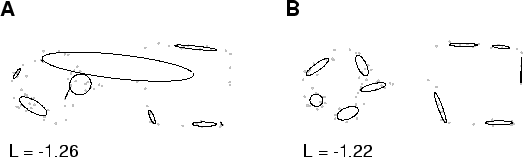Next: 3.3 Extension of the Up: 3.2 Extension of Neural Previous: 3.2.2 Alternative distance measure
Heiko Hoffmann
2005-03-22